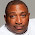## Friday, 20 January 2012

### T-SQL: Find missing gaps in data

I recently had solve a problem of finding missing values in a data series and at the same time, came across this article on the new Analytic Functions in SQL2012.

It turns out that there is a new function which can simplify my solution although as the problem is on a SQL2008 database, I won't be able to implement it. Still, another piece of the jigsaw.

Here, I'll present queries for each version:

`USE TempDBGO `

`-- setup the table `
`CREATE TABLE [dbo].[HoleyTable](   [Date] [datetime] NOT NULL,   [Country] [char](3) NOT NULL,   [Val] [decimal](22, 10) NULL) ON [PRIMARY]GO`

` -- and some sample data`
`INSERT INTO dbo.HoleyTable (Date, Country, Val) VALUES ('20100131','GBP', 40) `
`INSERT INTO dbo.HoleyTable (Date, Country, Val) VALUES ('20100331','GBP', 30) `
`INSERT INTO dbo.HoleyTable (Date, Country, Val) VALUES ('20100531','GBP', 20)`
`INSERT INTO dbo.HoleyTable (Date, Country, Val) VALUES ('20101031','GBP', 50) `
`INSERT INTO dbo.HoleyTable (Date, Country, Val) VALUES ('20101231','GBP', 55) `
`INSERT INTO dbo.HoleyTable (Date, Country, Val) VALUES ('20100228','USD', 20)`
`INSERT INTO dbo.HoleyTable (Date, Country, Val) VALUES ('20100331','USD', 10)`
`INSERT INTO dbo.HoleyTable (Date, Country, Val) VALUES ('20100430','USD', 15) `
`INSERT INTO dbo.HoleyTable (Date, Country, Val) VALUES ('20100630','USD', 25)`
`INSERT INTO dbo.HoleyTable (Date, Country, Val) VALUES ('20100731','USD', 55) `
`INSERT INTO dbo.HoleyTable (Date, Country, Val) VALUES ('20101130','USD', 30) `
`GO `

`-- SQL2008`
`WITH RankedData `
`AS`
`( `
`SELECT *, ROW_NUMBER() OVER (PARTITION BY Country ORDER BY Date) AS rn `
`FROM dbo.HoleyTable `
`) `
`SELECT a.Country,   b.Date AS StartOfGap,   a.Date AS EndOfGap,    DATEDIFF(m, b.date, a.date)-1 AS missingdatapoints `
`FROM RankedData a   INNER JOIN RankedData b       ON a.rn = b.rn + 1           AND a.Country = b.Country `
`WHERE DATEDIFF(m, b.date, a.date) > 1; `
`GO`

`-- SQL2012`
`WITH PeekAtData  `
`AS`
`(`
`SELECT *,        curr = date,       nxt = LEAD(Date, 1, NULL) OVER (PARTITION BY Country ORDER BY date)FROM dbo.HoleyTable `
`)`
`SELECT Country,      curr AS StartOfGap,       nxt AS EndOfGap,   DATEDIFF(m, curr, nxt) -1 AS MissingDatapoints `
`FROM PeekAtData p`
`WHERE DATEDIFF(m, curr, nxt) > 1GO `

`--tidy up`
`DROP TABLE dbo.HoleyTable`
`GO`

And how about the all important question of performance? Well, here is a screenshot of the execution plans for the 2 queries where you can see the 2012 implementation does outperform its 2008 counterpart by a ratio of about 3:1.

#### 1 comment:

1.Surprised there were no comments on this post. Very good!

/* add this crazy stuff in so i can use syntax highlighter